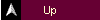Data Structures 1. Multi-value Functions 2. Lists and Streams 3. Descriptors 4. Trees

##### 18  EXTERNAL  INTERFACES

18.1 Foreign declarations

18.2 Calling Sequences

18.3 Strings in C

18.4 Arrays in C

18.5 Examples

The Elisa language provides facilities to acces external functions written in other programming languages. The current implementation of Elisa is restricted to external routines written in C.

18.1 Foreign declarations

A foreign declaration specifies an external function. The general form of a foreign declaration is:

`foreign(c) signature;`

The signature specifies the name, the number and the types of the parameters, and the result specification of the external function. The result specification may not contain the quantifiers optional and multi.

As an example, the following declaration:

`foreign(c) sin(real) -> real;`

specifies that the sin(real) -> real is an external C function.

A call in an Elisa program of

`sin(x)`

will execute the corresponding C function and will return the value of sin(x)

Other examples are:

`type double = subtype(real);`
```foreign(c) sin(double) -> real;
foreign(c) cos(double) -> real;
foreign(c) atan(double) -> real;
foreign(c) log(double) -> real;
foreign(c) exp(double) -> real;```

18.2 Calling Sequences

A C function has a calling sequence which is different from a calling sequence in Elisa. Also the implementation of arguments is different. Here is an overview of the basic data types as illustrated by the following table:

 Elisa C Storage character char 1 byte boolean not defined 1 byte integer long 4 bytes real double 8 bytes

As will be clear from this table: there are no major obstacles to map a basic data type from Elisa to C and visa versa. However, the memory layouts of strings, arrays and structures are quite different.

18.3 Strings in C

In the C language a string is an array of characters closed by a null character. In Elisa, text, which is an array of characters, can be converted to a C-string by means of the foreign function:

`foreign(c) _Cstring(text) -> CstringPtr;`

The _Cstring function converts an Elisa text into a pointer to a Cstring. The CstringPtr is defined by:

`type CstringPtr = subtype(integer);`

It is also possible to convert a Cstring into an Elisa text by means of the foreign function:

`foreign(c) _text(CstringPtr) -> text;`

The complete set of string conversion declarations is:

```    type CstringPtr = subtype(integer);
foreign(c) _Cstring(text) -> CstringPtr;
foreign(c) _text(CstringPtr) -> text;

```

18.4 Arrays in C

The memory layouts of Elisa arrays and C arrays are incompatible. In some foreign  C functions a reference to a C array is required. This can be done by converting an array written in Elisa to an array processable by C. For example, an array of reals in Elisa can be converted to a pointer to the array of reals as is used in C,  by means of the foreign function:

` foreign(c) _ArrayPtr(array(real)) -> CarrayPtr;`

The _ArrayPtr function converts  an Elisa array of reals into a pointer to a Carray. The CarrayPtr is defined by:

`type CarrayPtr = subtype(integer);`

Array conversion declarations are:

```    foreign(c) _ArrayPtr(array(character)) -> CarrayPtr;
foreign(c) _ArrayPtr(array(boolean)) -> CarrayPtr;
foreign(c) _ArrayPtr(array(integer)) -> CarrayPtr;
foreign(c) _ArrayPtr(array(real)) -> CarrayPtr;
```

The use of foreign C declarations to operate on C files are:

```type CstringPtr = subtype(integer);
foreign(c) _Cstring(text) -> CstringPtr;
foreign(c) _text(CstringPtr) -> text;

type CarrayPtr = subtype(integer);
foreign(c) _ArrayPtr(text) -> CarrayPtr;
type TextFile = subtype(integer);```
`type StreamFile = subtype(integer);`
```foreign(c) fopen(FileName = CstringPtr, FileMode = CstringPtr) -> TextFile;
foreign(c) fclose(TextFile) -> integer;
foreign(c) remove(FileName = CstringPtr) -> integer;

foreign(c) fgets(CstringPtr, MaxStringSize = integer, TextFile) -> integer;
foreign(c) strlen(CstringPtr) -> integer;

foreign(c) ObtainErrorFile( ) -> TextFile;
foreign(c) fprintf(outtextfile = TextFile, format = CstringPtr, character) -> nothing;

foreign(c) fseek(StreamFile, FileOffset = integer, Origin = integer) -> integer;
foreign(c) fwrite( CarrayPtr, Size = integer, Number = integer, StreamFile) -> integer;

```Part 1: Language Description Chapter 18: External Interfaces